# Set Linear Varied Total Head

The Set Linearly Varied Total Head option allows you to define Total Head boundary conditions which vary linearly along a boundary.

NOTE: see the Set Boundary Conditions topic for general information about defining groundwater boundary conditions for a finite element seepage analysis.

Linearly varying total head (0 to 5) defined on boundary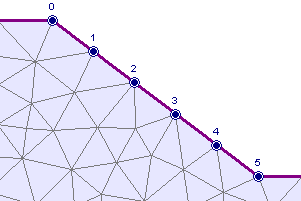To use this option:

2. You will see the Linearly Varied Total Head dialog:
• Choose the method of varying the Total Head along the boundary (Vertical Distance or Distance on Boundary). See below for more information.
• Enter the value of Total Head at Start and Total Head at End.
• If your model is staged, AND you wish to define different values of Total Head at different stages, select the Stage Total Heads check box and enter the desired values at each stage. select [OK].
3. Use the mouse to select the starting vertex and ending vertex on the desired boundary.
4. After the end vertex has been selected, the Linearly Varying Total Head boundary condition will be applied to the boundary, between the start and end vertices you have selected. The value of Total Head at each node of the mesh along the boundary will be displayed.

NOTE: the Total Head is calculated along the boundary according to the equations given below for each method (Vertical Distance or Distance on Boundary).

## Calculation of Total Head On Boundary

For the Linearly Varied Total Head option, the Total Head is calculated at each mesh node along the boundary, according to Equation 1.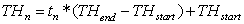Eqn.1

where: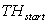is the Total Head at the start vertex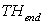is the Total Head at the end vertex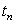is the relative distance of the mesh node along the boundary, calculated according to either Equation 2 or Equation 3, depending on the selected method (Vertical Distance or Distance on Boundary).

Vertical Distance Method

For the Vertical Distance method,is calculated using Equation 2.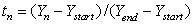Eqn.2

where: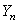is the vertical coordinate of the mesh node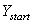is the vertical coordinate of the start vertex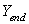is the vertical coordinate of the end vertex

NOTE: if the start and end vertices have the same Y-coordinate, then you cannot use the Vertical Distance option, you must use the Distance on Boundary option.

Distance on Boundary Method

For the Distance on Boundary method,is calculated using Equation 3.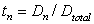Eqn.3

where: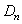is the distance along the boundary from the start vertex to the mesh node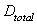is the total distance along the boundary from the start vertex to the end vertex.